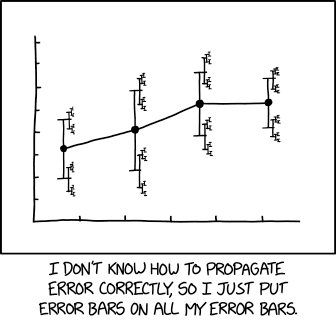# How can I estimate meta-uncertainty?

A type A uncertainty estimate is derived from repeated measurements. For example, I may estimate the uncertainty on a measurement by repeating the measurement $$N$$ times and then calculating some measure of its dispersion, such as the standard deviation or, as below, the Allan deviation (suitable when $$y$$ might vary slowly over time):

$$u_y = \sqrt{\frac{1}{2(N-1)} \sum_{i=1}^{N-1} (y_{i+1} - y_i)^2}$$

If circumstances prevent me from taking any more measurements, how can I use the distribution of $$y$$ to estimate the meta-uncertainty $$u_u$$, (i.e. the uncertainty of the uncertainty)?Source: XKCD

• I saw now this old question of yours. If you're still interested in the answer, I can provide it, with some references, also for the Allan deviation. It might take some time, but let me know. Jun 15, 2022 at 14:37
• @MassimoOrtolano Thanks for the offer. In reality, I have no practical need for an answer any more, as I am meanwhile working on a different topic. Jun 15, 2022 at 15:55
• Anyway I've bookmarked this and if I find the time I'll write an answer anyway since it's a very specialized topic, something I'm very fond of, and it might be useful to contribute to the spread of certain topics (I've already written a bit about the Allan variance in this answer). Jun 15, 2022 at 16:52